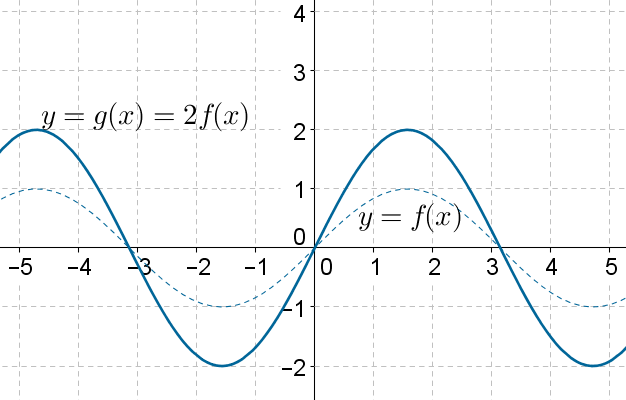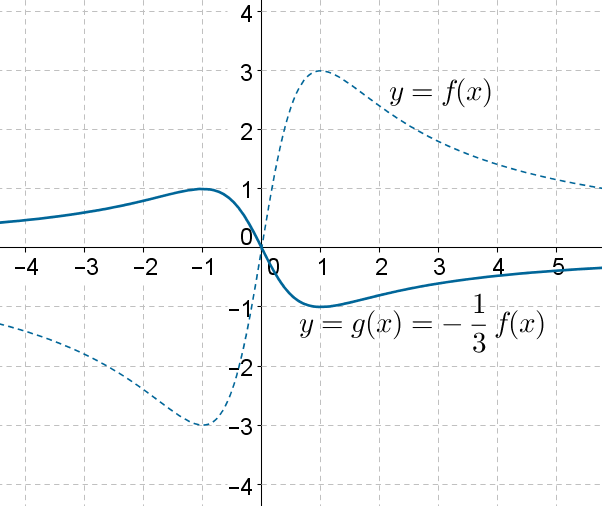# Vertical Scaling

Go back to  'Functions'

The following figure shows the graph of $$y = f\left( x \right)$$ (the dotted curve) where f is some function, and the graph of $$y = g\left( x \right) = 2 f\left( x \right)$$(the solid curve):What was the effect of multiplying by 2? The graph has vertically scaled by a factor of 2. All values have doubled; if $$\left( {{x_0},{y_0}} \right)$$is a point on the graph of f, then $$\left({{x_0},2{y_0}} \right)$$ is the corresponding point on the graph of g(note that the points where the graph crosses the x-axis, or the zeroes,are still the same – can you see why?).

The following figure shows the graph of $$y = f\left( x \right)$$ (the dotted curve) where f is some function, and the graph of $$y = g\left( x \right) = - \frac{1}{3}f\left( x\right)$$ (the solid curve):What has happened? Not only has the graph scaled by a factor of 1/3, it has flipped as well, because of the negative sign of the multiplier.

In general, the graph of $$y = kf\left( x \right)$$ can be obtained by vertically scaling the graph of $$y = f\left( x\right)$$ by $$\left| k \right|$$units. If k is negative, the graph will also flip. If the magnitude of is greater than 1, then the graph will stretch vertically,because each value will get scaled by a factor of magnitude greater than 1. If the magnitude of k is less than 1, then the graph will compress vertically, because each value will reduce in magnitude.

Functions
Functions
grade 10 | Questions Set 2
Functions
Functions
grade 10 | Questions Set 1